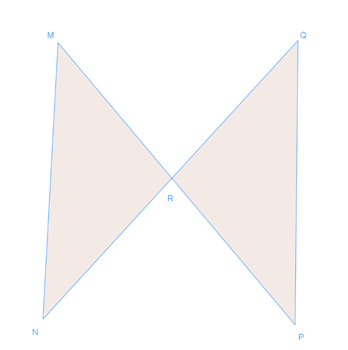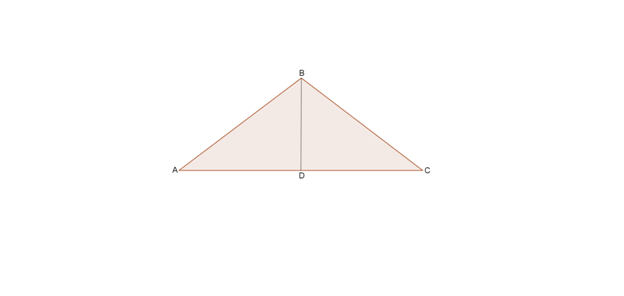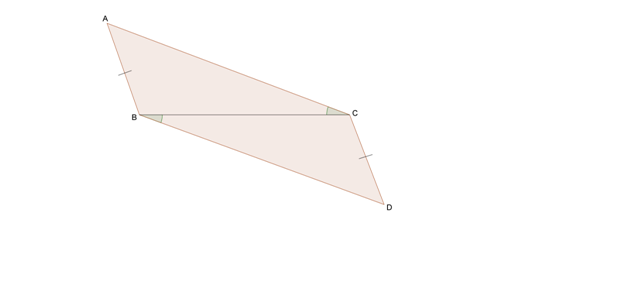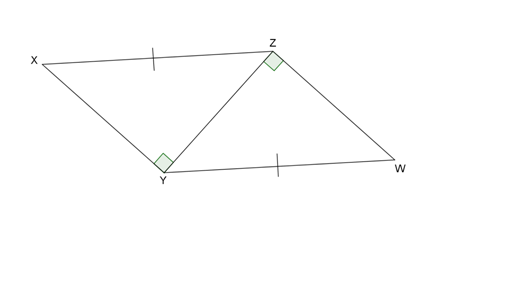# Triangles congruent by SAS and HL proofs#### Everything You Need in One Place

Homework problems? Exam preparation? Trying to grasp a concept or just brushing up the basics? Our extensive help & practice library have got you covered.#### Learn and Practice With Ease

Our proven video lessons ease you through problems quickly, and you get tonnes of friendly practice on questions that trip students up on tests and finals.#### Instant and Unlimited Help

Our personalized learning platform enables you to instantly find the exact walkthrough to your specific type of question. Activate unlimited help now!

0/1
##### Intros
###### Lessons
1. Similar tirangles VS. Congruent triangles
2. Ways to prove congruency:
• SSS
• SAS
• ASA
• AAS
0/6
##### Examples
###### Lessons
1. Write a two-column proof.
1. Given: R is the midpoint of $\overline {MP}$.
R is the midpoint of $\overline {NQ}$.
Prove: $\triangle MRN$$\cong$$\triangle PRQ$2. Special case for right triangle:
Given: $\triangle ABC$ is an isosceles triangle.
D is the midpoint of $\overline {AC}$.
Prove: $\triangle ABD$$\cong$$\triangle CBD$.2. Write a flow proof
1. Given: R is the midpoint of $\overline {MP}$.
R is the midpoint of $\overline {NQ}$.
Prove: $\triangle MRN$$\cong$$\triangle PRQ$2. Special case for right triangle:
Given: $\triangle ABC$ is an isosceles triangle.
D is the midpoint of $\overline {AC}$.
Prove: $\triangle ABD$$\cong$$\triangle CBD$.3. Is there enough information given to prove the following triangles are congruent? If so, explain your reasoning and point out the theorem or postulate you use.

1.2.